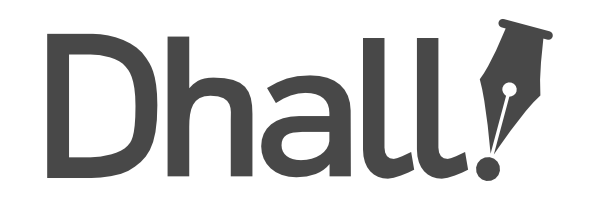# Are there type variables / generic types?

#1

Hi, just starting with Dhall. I will like to do something like this:

``````let (Thing a) : Type =
{ output : a
, a : Text
, b : Text
}

let stringThings : List (Thing Text) =
[ {output = "out", a= "a", b = "b"}
, {output = "out", a= "a", b = "b"}
]

let intThings : List (Thing Integer) =
[ {output = 1, a= "a",  b = "b"}
, {output = 1,  a= "a", b = "b"}
]
``````

So I would like to have a list of types where one or two attributes are generic.
Is there such a thing in Dhall?

One way I can think of is to create different concrete types:

``````let TextThing : Type
let IntThing: Type
let DoubleThing : Type
let OptionalTextThing : Type
...
``````

But this will create a lot of repetition.

Another way is to use a union in the `output`, But then elements in the list could have mixed outputs. I want all elements in a list to have the same output.

Any other way to achieve something like this?

#2

Perhaps you can achieve that with a function to create the type, for example:

``````let Thing = λ(a : Type) → { output : a, a : Text, b : Text }

let stringThings
: List (Thing Text)
= [ { output = "out", a = "a", b = "b" }
, { output = "out", a = "a", b = "b" }
]

let intThings
: List (Thing Integer)
= [ { output = +1, a = "a", b = "b" }, { output = +1, a = "a", b = "b" } ]

let Thing/getA = λ(a : Type) → λ(thing : Thing a) → thing.a

in  Thing/getA Text { output = "out", a = "a", b = "b" }
``````

#3

Nice, this works really well, thanks.